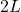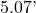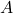In remembrance of Dr. Terry Kohutek, Texas A & M, Professor of Civil Engineering
Well-respected teacher

https://www.dignitymemorial.com/obituaries/college-station-tx/terry-kohutek-5194499

Many years ago (1989, a third of a century ago), I was in an oral exam at TAMU; several civil engineering faculty were in the exam room.

Dr. Kohutek asked about a pin-supported frame subjected to a single concentrated vertical load:• The frame itself is symmetric.
• Consider, he said, the load to be over the center of the beam; no sidesway deflection is expected — symmetry.
• Next he said, consider the load to be over the left column; no sidesway deflection is expected (due to flexural deformation).
• Next he said, consider the load to be over the right column; again no sidesway deflection is expected (again, due to flexure deformation).
• Now, place the load just to the right of the left column.
What is the expected horizontal displacement for this loading?
Which direction is the horizontal deflection?

I answered — incorrectly — that the deflection would be toward the left. Dr. Kohutek said the deflection would be toward the right. There was a vigorous discussion with more faculty saying left than right.

After the exam, Kohutek checked the answer using RISA (the first time I ever heard of the software). Of course, he was correct, it was his question.

I’ve been a bit bothered all these years, that I missed a question that was fairly easy to analyze but bothered also that the wrong answer seemed to prevail within the exam room (*). It certainly must have annoyed Dr. Kohutek.

In the PDF link, I present complete calculations:

• Calculation of the horizontal reactions at the base of the columns
• Calculation of the horizontal displacement at the beam level
• First, I determine the base reactionsandusing the method of consistent deflections
• Then I solve forusing the method of virtual work.
• In this specific example:
The column length isand the beam length isAll members are W8x24 steel beams
I place the 1 kip vertical load,, at one-sixth of the beam length.
• I also offer a graphical proof that the deflection is to the right.
See pages 17 through 20.
• See the “Further Derivation” below for a closed form solution forsuch thatis maximum.
• See the “General Solution” below for a closed form solution forFor any frame geometry.
• As Vern Gosdin said, “That just about does it, don’t it”

Click for Influence Line

Of course, he was correct, it was his question.

Comparison of horizontal displacement results:

 My hand calculation Δ = 0.0384 inches RISA-3D Δ = 0.0387 inches SAP2000 Δ = 0.0383 inches
Further Derivation
For the problem stated on Page 1 of the calculations, keepingas a variable,
I derivedin terms of:Calculating, and solving forwhereis a maximum or minimum:Using thevalue:Remember, in our problem,is the height of the column, given in feetTherefore,, which occurs whenis located at. These values are in agreement with the influence diagram determined using RISA-3D.
General Solution
Ultimately, I decided to develop a general solution. Below I present the general sketch, general parameters, and the general derivation. I also offer calculations for a specific example to allow comparison with commercial structural software.

In this general solution:

• First, I use the method of consistent deflections to determine the base reactionsand• Then I use Castigliano’s 2nd Theorem, to solve for.The parameters are:

Parameter Example Value: length of the beam 24 feet: length of the two columns in the bent frame varies: distance from left support to point load 4 feet: distance from right support to point load 20 feet: A/L 1/6: B/L 5/6: moment of inertia of beam I=307 for W12x40: moment of inertia of columns φ=1: area of beam A=11.7 for W12x40: area of columns Θ=1

Click for General Formula calculations

Formula for deflection due to bending deformation in Cell 23Formula for deflection due to axial deformation in Cell 30When theload is centered (at), both formulas go to zero, as expected for a symmetric loading. What might not be expected, is that a horizontal displacement is generated byloadings at the column lines (). This horizontal displacement is solely the result of axial deformation in the columns.

Click for Tabular Results for the Example
For completeness
For completeness, here are three LIVE graphs:

Axial deflection along the beam

Bending deflection along the beam

Total deflection (axial + bending) along the beam

In the graphs, notice that there are 3 points specially marked along the beam; these represent the 3 alpha locations resulting in. Refer to the general formula calculations.

In this specific example:The column length isand the beam length isTherefore, the column length is 12′ and the beam length is 24′

All members are W8x24 steel beams

(*) I guess we have all seen this happen in meetings.